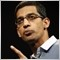# Find the highest value of an indicator in a period X - page 2696

WHRoeder:

 int ArrayMaximum( double array[], int count=WHOLE_ARRAY, int start=0)

Searches for the element with maximum value. The function returns position of this maximum element in the array.

It will be the index of the element, not the element itself.355

rodrigosm:

zzuegg, thanks for the help. I'm beginner, but I'll study how to do that you wrote. I think the first one is easier, but I still not knowing how to implement this in my formula. If possible, give me an example.

Thanks again.

i think option 2 is easier. He already gave you the pseudocode you need. just replace the function header, put in some brackets, and replace RSI(i) with the iRSI function. Pretty straightforward and there's no need to mess with additional arrays. This is how i would do it.362

hasayama:
 int ArrayMaximum( double array[], int count=WHOLE_ARRAY, int start=0)

Searches for the element with maximum value. The function returns position of this maximum element in the array.

It will be the index of the element, not the element itself.

Yeah, I tested it and did not work.25339

hasayama:
 int ArrayMaximum( double array[], int count=WHOLE_ARRAY, int start=0)

Searches for the element with maximum value. The function returns position of this maximum element in the array.

It will be the index of the element, not the element itself.

So think for a change
```for(int i = limit-1; i>=0; i--) // Count down for one pass
RSI_Buffer[i]=iRSI(NULL,0,periodoRSI,PRICE_CLOSE,i);
//   a1_Buffer[i] = ArrayMaximum(RSI_Buffer, 20, i);
a1_Buffer[i] = RSI_Buffer[ArrayMaximum(RSI_Buffer, 20, i)];```362

WHRoeder:
So think for a change

Thanks... worked very well this change! I didn't know that it was allowable.

`a1_Buffer[i] = RSI_Buffer[ArrayMaximum(RSI_Buffer, 20, i)];`

Great job. I will finish the indicator and post here.362

Thanks everybody! I finished the indicator.

```//+------------------------------------------------------------------+
//|                                                  Divergência.mq4|
//|                                        http://www.metaquotes.net |
//+------------------------------------------------------------------+

#property indicator_separate_window
#property indicator_minimum -1
#property indicator_maximum +1
#property indicator_buffers 2
#property indicator_color1 Red
#property indicator_color2 LawnGreen
//-Parametros
extern int periodo=20;
extern int periodoRSI=20;

//---- buffers
double ExtMapBuffer1[];
double ExtMapBuffer2[];
double RSI_Buffer[];
double RSI_Buffer2[];
double a1_Buffer[];
double a2_Buffer[];
double b1_Buffer[];
double b2_Buffer[];
//+------------------------------------------------------------------+
//| Custom indicator initialization function                         |
//+------------------------------------------------------------------+
int init()
{
//---- indicators
IndicatorBuffers(8);
//--- Internal Variables

SetIndexBuffer(2,RSI_Buffer);
SetIndexBuffer(3,a1_Buffer);
SetIndexBuffer(4,a2_Buffer);
SetIndexBuffer(5,b1_Buffer);
SetIndexBuffer(6,b2_Buffer);
SetIndexBuffer(7,RSI_Buffer2);

//--- Plot a line
SetIndexStyle(0,DRAW_LINE);
SetIndexBuffer(0,ExtMapBuffer1);
SetIndexStyle(1,DRAW_LINE);
SetIndexBuffer(1,ExtMapBuffer2);
//----
return(0);
}
//+------------------------------------------------------------------+
//| Custom indicator deinitialization function                       |
//+------------------------------------------------------------------+
int deinit()
{
//----

//----
return(0);
}
//+------------------------------------------------------------------+
//| Custom indicator iteration function                              |
//+------------------------------------------------------------------+

int start()
{

//---- last counted bar will be recounted
int counted_bars = IndicatorCounted();
if(counted_bars>0) counted_bars--;
int limit=Bars-counted_bars;

//---- Current RSI value
for(int i = limit-1; i>=0; i--)// Count down for one pass
RSI_Buffer[i]=iRSI(NULL,0,periodoRSI,PRICE_CLOSE,i);
//---- Highest price in 20 periods
for(i = limit-1; i>=0; i--)// Count down for one pass
a1_Buffer[i]=High[iHighest(NULL,0,MODE_HIGH,periodo,i)];
//---- Highest RSI value in 20 periods
for(i = limit-1; i>=0; i--) // Count down for one pass
a2_Buffer[i] = RSI_Buffer[ArrayMaximum(RSI_Buffer, periodo, i)];
//---- Lowest price in 20 periods
for(i = 0; i<limit; i++)
b1_Buffer[i]=Low[iLowest(NULL,0,MODE_LOW,periodo,i)];
//---- Lowest RSI value in 20 periods
for(i= limit-1; i>=0; i--)
b2_Buffer[i] = RSI_Buffer[ArrayMinimum(RSI_Buffer, periodo, i)];

//---- Bullish Divergence
for(i= limit-1; i>=0; i--)
{
if((Close[i]<=b1_Buffer[i] && RSI_Buffer[i]>b2_Buffer[i]) || (RSI_Buffer[i]<=b2_Buffer[i] && Close[i]>b1_Buffer[i]))
ExtMapBuffer1[i]=-1;
else
ExtMapBuffer1[i]=0;

}

//---  Bearish Divergence
for(i= limit-1; i>=0; i--)
{
if((Close[i]>=a1_Buffer[i] && RSI_Buffer[i]<a2_Buffer[i])|| (RSI_Buffer[i]>=a2_Buffer[i] && Close[i]<a1_Buffer[i]))
ExtMapBuffer2[i]=1;
else
ExtMapBuffer2[i]=0;

}

//----
return(0);
}
//+------------------------------------------------------------------+```2207

hi i need a code for EA based mainly not for indiactor or script only for EA code

RSI for

and

RSI<20 means SELL

am new to mt4.so plss help me frnds..... thank u all[Deleted]

Hi guys,

I am a newbie and I need a code to determine the value of RSI at the end of each period (at the end of M1, M5, ....)17401

newcoder:

Hi guys,

I am a newbie and I need a code to determine the value of RSI at the end of each period (at the end of M1, M5, ....)